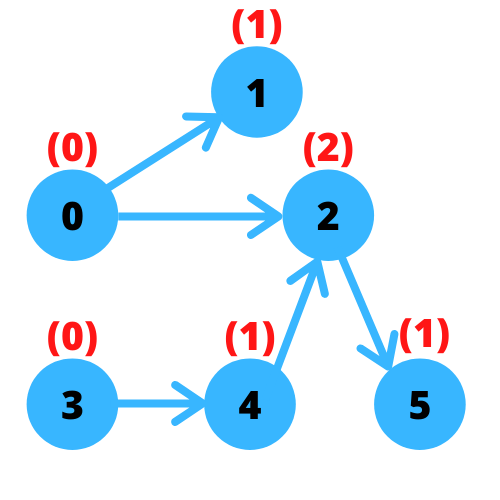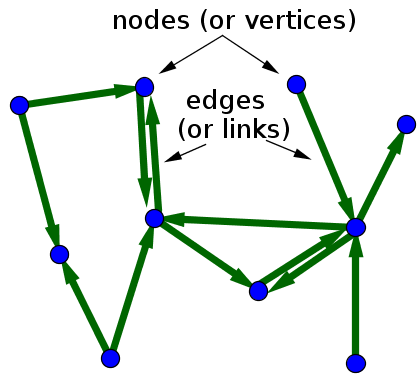# Minimum Number of Vertices to Reach All Nodes By Graph Theory With Python and JavascriptMinimum Number of Vertices to Reach All Nodes By Graph Theory With Python and Javascript. Solving a medium leetcode problem with graph theory

## Understanding the Problem

Minimum Number of Vertices to Reach All Nodes is a medium problem at leetcode, I hope you have read the problem carefully and have tried solving it. This problem requires you to get the least number of vertices that can make you pass all nodes you have for the graph of your problem. The question is what are these vertices?

## Why graph theory?

You’re given an array of arrays (or a list of lists in a pythonic way) and each subarray contains two numbers entailing the first one to be the node from which you can move and the other one is the node to which you reach. These nodes are called vertices and each path connecting them is called an edge, the whole picture is a graph. In mathematics, graph theory is a study of such graphs and in this problem, we have a specific type of graph called directed graph where edges are connecting vertices asymmetrically meaning all edges are connecting from one vertex to another as you can see from the arrows in the image below:A directed graph with 10 vertices and 13 edges (source)

How graph works in this problem?

To know how it works, we need to know one thing: in-degree. Let’s see what that means through these examples

## Guide to Python Programming Language

Guide to Python Programming Language

## Minimum Numbers of Function Calls to Make Target Array With Python and Javascript

Minimum Numbers of Function Calls to Make Target Array With Python and Javascript. With Python and Javascript solutions: Solving a medium leetcode problem

## Python Programming: A Beginner’s Guide

Python is an interpreted, high-level, powerful general-purpose programming language. You may ask, Python’s a snake right? and Why is this programming language named after it?

## Python Hello World Program - Create & Run Your First Python Program in PyCharm

Python Hello World Program - Your first step towards Python world. Learn how to create the Hello World Python program in PyCharm.

## Python Programming Tutorials For Beginners

Python Programming Tutorials For Beginners